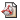## Learning Algorithms

Neural networks and other statistical pattern recognition methods learn a task on the basis of experience, that is to say from data. Machine learning is a very broad field and contains many subtopics. At SNN Nijmegen we have addressed a number of these topics. These are summarized on this page.

We study the problem of multi-task learning in a Bayesian setting. Rather than having to learn a single task, there are a huge number of similar tasks. The challenge is to let these tasks "learn from each other". The Bayesian machinery comes in when we start from the prior that the weights associated with each task should be similar and compute the posterior distribution given the data over all tasks. We have applied this idea to the prediction of single-copy newspaper sales where each individual task corresponds to prediction of a single outlet.

### Survival analysis

The problem of survival analysis is to predict the survival of a group of patients in a future time window based on their symptoms and characteristics. The most popular methodology in this field is Cox proportional hazards. This method can be given a neural interpretation, in which the priors for the model come rather naturally and which can be generalized to allow for more complex relationships. Typical of these kinds of problems is the large amount of (possible) explanatory variables with the risk of over-fitting. We demonstrate that the neural-Bayesian framework reduces the risk of over-fitting in an elegant manner and that variational techniques can be used to approximate the posterior distribution over model parameters efficiently.

### On-line Learning

With on-line learning, network weights are updated at the presentation of each training pattern. Usually, these training patterns are drawn at random from the set of all training patterns. This introduces a kind of stochasticity. Understanding and exploiting this stochasticity forms the basis of most of our theoretical work on on-line learning processes. We studied how this noise can help to escape local minima and to speed up learning on plateaus. We designed schedules for tuning the learning parameter, both for learning in changing environments and for global optimization. At a later stage we looked at the use of momentum terms in on-line learning and the effect of correlations between subsequently presented patterns, the latter both for "small" and "large" networks. Our most recent work concerns natural gradient on-line learning.

### Self-Organizing Maps

Self-organizing maps are popular tools for clustering and visualization of high-dimensional data. Kohonen's original algorithm is still very popular: it is easy to write down, to simulate, to understand (at least at a basic level), and has many important applications. One of the main arguments against it, is the lack of a solid theoretical basis. For that reason, we have slightly adapted the original definition by changing the determination of the best matching unit. Then the Kohonen learning rule can be derived from an energy function, on which it performs (stochastic) gradient descent. The existence of this energy function enables us to study global convergence properties and develop new applications. Exploiting the link between self-organizing maps and mixture modeling, we have derived fast EM-algorithms.

### Confidence Levels in Neural Networks

Neural networks offer a general tool for classification and regression. For regression tasks, a neural network output can be interpreted as an estimate of the average output given a particular input, for classification the probability that a particular input belongs to some class. A natural extension is to provide also an estimate of the confidence to these outputs, such as error bars for regression and probability of misclassification for classification problems. The emphasis has been on regression problems, which have been tackled mainly using frequentist techniques (bootstrapping), but also following Bayesian procedures (HMCMC sampling as well as variational techniques). In both cases, we have to deal with an ensemble of neural networks rather than a single network. The variation within the ensemble yields a measure of confidence which can be translated into error bars. More theoretical work deals with the derivation of bias-variance decompositions. The software package NetPack implements the result of this research.

### Input Relevance and feature selection

The usual approach in neural network modeling is to include all input variables that might have an effect on the output. Incorporation of too many inputs, however, degrades the generalization performance of the model. Furthermore, a model with irrelevant variables is much more difficult to interpret than a smaller one, only taking into account the relevant inputs. We have developed methods for determining the relevance of input variables and thus to select the most relevant features. To really measure the relevance of an input, one should compare a neural network trained with and without this particular input. This soon becomes very time-consuming and inefficient. The basic idea behind our algorithm called "partial retraining" is to approximate this full retraining process by fitting the model layer by layer. Partial retraining is faster and more robust than other suggestions. It can trivially be expanded to the pruning of individual network weights rather than whole input units. The software package NetPack implements some of the result of this research.

### Related Articles

Duane G.S., Wiegerinck W.A.J.J., Selten F., Shen M.L, Keenlyside N.
Supermodeling: synchronization of alternative dynamical models of a single objective process .
Advanced in Nonlinear Geosciences, pp. 101-121, 2018

Kappen H.J.
Learning quantum models from quantum or classical data.
arxiv, vol. 11278, 2018Wiegerinck W.A.J.J., Selten F.
Attractor learning in synchronized chaotic systems in the presence of unresolved scales.
Chaos, vol. 27, no. 126901, pp. 1-37, 2017

Duane G.S., Wiegerinck W.A.J.J., Selten F., Shen M.L, Keenlyside N.
Supermodeling: synchronization of alternative dynamical models of a single objective process.
Advanced in Nonlinear Geosciences, pp. 101-121, 2017Thijssen S.A., Kappen H.J.
Path integral control and state dependent feedback.
Physical Review E, vol. 91, no. 032104, pp. 1-6, 2015

All SNN publications